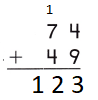Refer to our Texas Go Math Grade 2 Answer Key Pdf to score good marks in the exams. Test yourself by practicing the problems from Texas Go Math Grade 2 Lesson 7.2 Answer Key Practice 2-Digit Addition.

Explore

Choose a tool for solving the problem. You could use real objects, blocks, or paper and pencil. Then draw or write to show what you did.

FOR THE TEACHER • Read the following problem. There were 27 boys and 18 girls who ran in the race. How many children ran in the race?
45 children
Explanation:
There were 27 boys and 18 girls who ran in the race.
27 + 18 = 45
45 children ran in the race

Math Talk
Mathematical Processes

Explain your choice for solving the problem.
Explanation:
For solving the above problem we have to add the number of girls and boys

Model and Draw

Mrs. Meyers sold 47 snacks before the game. Then she sold 85 snacks during the game. How many snacks did she sell?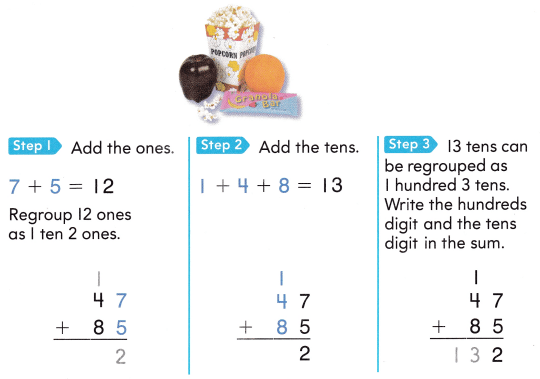Share and Show

Write the sum.

Question 1.Explanation:
regrouped the sum as tens and ones
the carry is added to the tens
then the tens are regrouped as tens and hundreds

Question 2.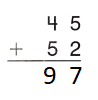Explanation:
regrouped the sum as tens and ones
the carry is added to the tens
then the tens are regrouped as tens and hundreds

Question 3.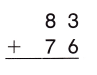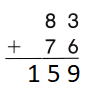Explanation:
regrouped the sum as tens and ones
the carry is added to the tens
then the tens are regrouped as tens and hundreds

Question 4.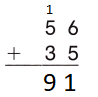Explanation:
regrouped the sum as tens and ones
the carry is added to the tens
then the tens are regrouped as tens and hundreds

Question 5.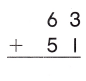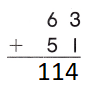Explanation:
regrouped the sum as tens and ones
the carry is added to the tens
then the tens are regrouped as tens and hundreds

Question 6.